# 20.04: Naming Alkanes

$$\newcommand{\vecs}{\overset { \rightharpoonup} {\mathbf{#1}} }$$ $$\newcommand{\vecd}{\overset{-\!-\!\rightharpoonup}{\vphantom{a}\smash {#1}}}$$$$\newcommand{\id}{\mathrm{id}}$$ $$\newcommand{\Span}{\mathrm{span}}$$ $$\newcommand{\kernel}{\mathrm{null}\,}$$ $$\newcommand{\range}{\mathrm{range}\,}$$ $$\newcommand{\RealPart}{\mathrm{Re}}$$ $$\newcommand{\ImaginaryPart}{\mathrm{Im}}$$ $$\newcommand{\Argument}{\mathrm{Arg}}$$ $$\newcommand{\norm}{\| #1 \|}$$ $$\newcommand{\inner}{\langle #1, #2 \rangle}$$ $$\newcommand{\Span}{\mathrm{span}}$$ $$\newcommand{\id}{\mathrm{id}}$$ $$\newcommand{\Span}{\mathrm{span}}$$ $$\newcommand{\kernel}{\mathrm{null}\,}$$ $$\newcommand{\range}{\mathrm{range}\,}$$ $$\newcommand{\RealPart}{\mathrm{Re}}$$ $$\newcommand{\ImaginaryPart}{\mathrm{Im}}$$ $$\newcommand{\Argument}{\mathrm{Arg}}$$ $$\newcommand{\norm}{\| #1 \|}$$ $$\newcommand{\inner}{\langle #1, #2 \rangle}$$ $$\newcommand{\Span}{\mathrm{span}}$$$$\newcommand{\AA}{\unicode[.8,0]{x212B}}$$

Learning Objectives

• To identify and name simple (straight-chain) alkanes given formulas and write formulas for straight-chain alkanes given their names.
• To name branched chain alkanes by the IUPAC system and write formulas for branched chain alkanes given IUPAC names

We begin our study of organic chemistry with the hydrocarbons, the simplest organic compounds, which are composed of carbon and hydrogen atoms only. As we noted, there are several different kinds of hydrocarbons. They are distinguished by the types of bonding between carbon atoms and the properties that result from that bonding. Hydrocarbons with only carbon-to-carbon single bonds (C–C) and existing as a continuous chain of carbon atoms also bonded to hydrogen atoms are called alkanes (or saturated hydrocarbons). Saturated, in this case, means that each carbon atom is bonded to four other atoms (hydrogen or carbon)—the most possible; there are no double or triple bonds in the molecules.

The word saturated has the same meaning for hydrocarbons as it does for the dietary fats and oils: the molecule has no carbon-to-carbon double bonds (C=C).

We previously introduced the three simplest alkanes—methane (CH4), ethane (C2H6), and propane (C3H8) and they are shown again in Figure $$\PageIndex{1}$$.Figure $$\PageIndex{1}$$: The Three Simplest Alkanes

The flat representations shown do not accurately portray bond angles or molecular geometry. Methane has a tetrahedral shape that chemists often portray with wedges indicating bonds coming out toward you and dashed lines indicating bonds that go back away from you. An ordinary solid line indicates a bond in the plane of the page. Recall that the VSEPR theory correctly predicts a tetrahedral shape for the methane molecule (Figure $$\PageIndex{2}$$).Figure $$\PageIndex{2}$$: The Tetrahedral Methane Molecule

Methane (CH4), ethane (C2H6), and propane (C3H8) are the beginning of a series of compounds in which any two members in a sequence differ by one carbon atom and two hydrogen atoms—namely, a CH2 unit. The first 10 members of this series are given in Table $$\PageIndex{1}$$.

Table $$\PageIndex{1}$$: The First 10 Straight-Chain Alkanes
Name Molecular Formula (CnH2n + 2) Condensed Structural Formula Number of Possible Isomers
methane CH4 CH4
ethane C2H6 CH3CH3
propane C3H8 CH3CH2CH3
butane C4H10 CH3CH2CH2CH3 2
pentane C5H12 CH3CH2CH2CH2CH3 3
hexane C6H14 CH3CH2CH2CH2CH2CH3 5
heptane C7H16 CH3CH2CH2CH2CH2CH2CH3 9
octane C8H18 CH3CH2CH2CH2CH2CH2CH2CH3 18
nonane C9H20 CH3CH2CH2CH2CH2CH2CH2CH2CH3 35
decane C10H22 CH3CH2CH2CH2CH2CH2CH2CH2CH2CH3 75

Consider the series in Figure $$\PageIndex{3}$$. The sequence starts with C3H8, and a CH2 unit is added in each step moving up the series. Any family of compounds in which adjacent members differ from each other by a definite factor (here a CH2 group) is called a homologous series. The members of such a series, called homologs, have properties that vary in a regular and predictable manner. The principle of homology gives organization to organic chemistry in much the same way that the periodic table gives organization to inorganic chemistry. Instead of a bewildering array of individual carbon compounds, we can study a few members of a homologous series and from them deduce some of the properties of other compounds in the series.Figure $$\PageIndex{3}$$: Members of a Homologous Series. Each succeeding formula incorporates one carbon atom and two hydrogen atoms more than the previous formula.

The principle of homology allows us to write a general formula for alkanes: CnH2n + 2. Using this formula, we can write a molecular formula for any alkane with a given number of carbon atoms. For example, an alkane with eight carbon atoms has the molecular formula C8H(2 × 8) + 2 = C8H18.

## Branched Chain Alkanes

As noted in previously, the number of isomers increases rapidly as the number of carbon atoms increases. There are 3 pentanes, 5 hexanes, 9 heptanes, and 18 octanes. It would be difficult to assign unique individual names that we could remember. A systematic way of naming hydrocarbons and other organic compounds has been devised by the International Union of Pure and Applied Chemistry (IUPAC). These rules, used worldwide, are known as the IUPAC System of Nomenclature. (Some of the names we used earlier, such as isobutane, isopentane, and neopentane, do not follow these rules and are called common names.) A stem name (Table $$\PageIndex{1}$$) indicates the number of carbon atoms in the longest continuous chain (LCC). Atoms or groups attached to this carbon chain, called substituents, are then named, with their positions indicated by numbers. For now, we will consider only those substituents called alkyl groups.

Table $$\PageIndex{1}$$: Stems That Indicate the Number of Carbon Atoms in Organic Molecules
Stem Number
meth- 1
eth- 2
prop- 3
but- 4
pent- 5
hex- 6
hept- 7
oct- 8
non- 9
dec- 10

An alkyl group is a group of atoms that results when one hydrogen atom is removed from an alkane. The group is named by replacing the -ane suffix of the parent hydrocarbon with -yl. For example, the -CH3 group derived from methane (CH4) results from subtracting one hydrogen atom and is called a methyl group. The alkyl groups we will use most frequently are listed in Table $$\PageIndex{2}$$. Alkyl groups are not independent molecules; they are parts of molecules that we consider as a unit to name compounds systematically.

Table $$\PageIndex{2}$$: Common Alkyl Groups
Parent Alkane Alkyl Group Condensed Structural Formula
methanemethylCH3
ethane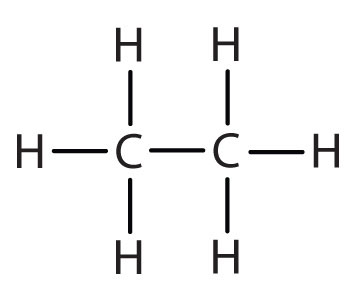ethyl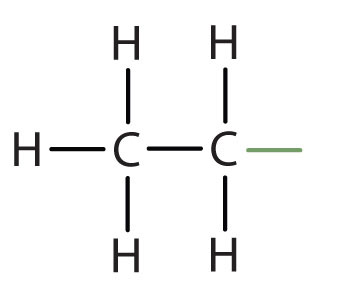CH3CH2
propane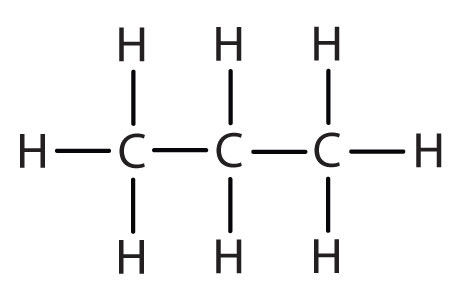propylCH3CH2CH2
isopropyl(CH3)2CH–
butanebutylCH3CH2CH2CH2
sec-butyl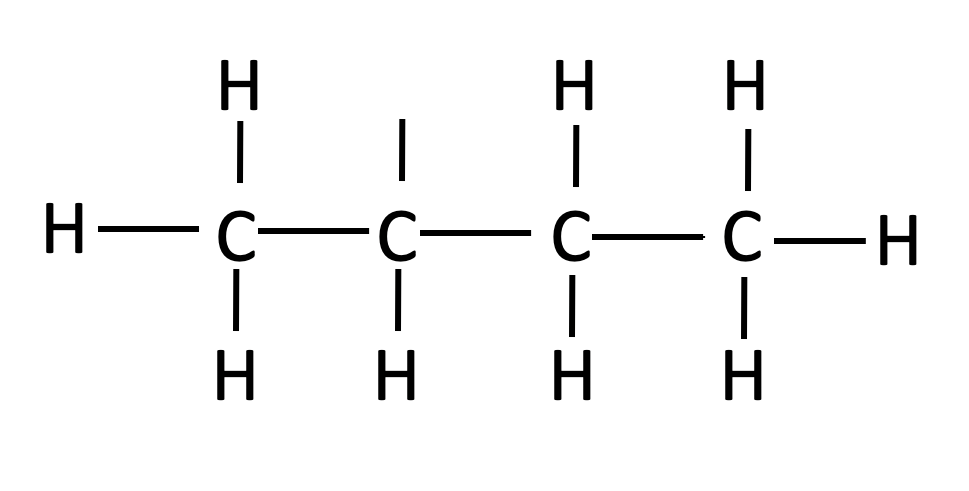CH3CH2(CH3)CH-
isobutyl-CH2CH(CH3)2
tert-butyl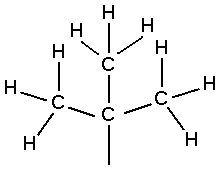-C(CH3)3

Simplified IUPAC rules for naming alkanes are as follows (demonstrated in Example $$\PageIndex{1}$$).

1. Name alkanes according to the LCC (longest continuous chain) of carbon atoms in the molecule (rather than the total number of carbon atoms). This LCC, considered the parent chain, determines the base name, to which we add the suffix -ane to indicate that the molecule is an alkane.

2. If the hydrocarbon is branched, number the carbon atoms of the LCC. Numbers are assigned in the direction that gives the lowest numbers to the carbon atoms with attached substituents. Hyphens are used to separate numbers from the names of substituents; commas separate numbers from each other. (The LCC need not be written in a straight line; for example, the LCC in the following has five carbon atoms.)3. Place the names of the substituent groups in alphabetical order before the name of the parent compound. If the same alkyl group appears more than once, the numbers of all the carbon atoms to which it is attached are expressed. If the same group appears more than once on the same carbon atom, the number of that carbon atom is repeated as many times as the group appears. Moreover, the number of identical groups is indicated by the Greek prefixes di-, tri-, tetra-, and so on. These prefixes are not considered in determining the alphabetical order of the substituents. For example, ethyl is listed before dimethyl; the di- is simply ignored. The last alkyl group named is prefixed to the name of the parent alkane to form one word.

When these rules are followed, every unique compound receives its own exclusive name. The rules enable us to not only name a compound from a given structure but also draw a structure from a given name. The best way to learn how to use the IUPAC system is to put it to work, not just memorize the rules. It’s easier than it looks.

Example $$\PageIndex{1}$$

Name each compound.

1.2.3.SOLUTION

1. The LCC has five carbon atoms, and so the parent compound is pentane (rule 1). There is a methyl group (rule 2) attached to the second carbon atom of the pentane chain. The name is therefore 2-methylpentane.
2. The LCC has six carbon atoms, so the parent compound is hexane (rule 1). Methyl groups (rule 2) are attached to the second and fifth carbon atoms. The name is 2,5-dimethylhexane.
3. The LCC has eight carbon atoms, so the parent compound is octane (rule 1). There are methyl and ethyl groups (rule 2), both attached to the fourth carbon atom (counting from the right gives this carbon atom a lower number; rule 3). The correct name is thus 4-ethyl-4-methyloctane.

Exercise $$\PageIndex{1}$$

Name each compound.

1.2.3.Example $$\PageIndex{2}$$

Draw the structure for each compound.

1. 2,3-dimethylbutane
2. 4-ethyl-2-methylheptane

SOLUTION

1. The parent chain is butane, indicating four carbon atoms in the LCC.Then add the groups at their proper positions. You can number the parent chain from either direction as long as you are consistent; just don’t change directions before the structure is done. The name indicates two methyl (CH3) groups, one on the second carbon atom and one on the third.Finally, fill in all the hydrogen atoms, keeping in mind that each carbon atom must have four bonds.2. The parent chain is heptane in this case, indicating seven carbon atoms in the LCC.

–C–C–C–C–C–C–C–

Adding the groups at their proper positions givesFilling in all the hydrogen atoms gives the following condensed structural formulas:Note that the bonds (dashes) can be shown or not; sometimes they are needed for spacing.

Exercise $$\PageIndex{2}$$

Draw the structure for each compound.

1. 4-ethyloctane
2. 3-ethyl-2-methylpentane
3. 3,3,5-trimethylheptane

## Key Takeaway

• Alkanes have both common names and systematic names, specified by IUPAC.